Mathematical and Physical Journal
for High Schools
Issued by the MATFUND Foundation
 Already signed up? New to KöMaL?

# KöMaL Problems in Physics, March 2015

Show/hide problems of signs:## Problems with sign 'M'

Deadline expired on April 10, 2015.

M. 349. A roll of cello-tape (or any other type of transparent adhesive tape) is placed to a fixed horizontal axle. The end of the tape is pulled vertically downward at some particular magnitude of force. Investigate how the tape rolls down (or breaks) at different magnitude of forces. Find the relationship between the average roll-off speed and the force.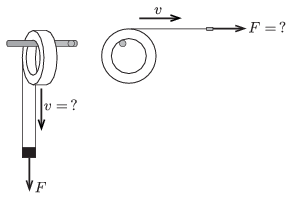Another type of measurement can also be chosen: tear off the tape at some given speed and investigate the average force applied. (Only one of the two different measurements should be carried out.)

(6 pont)

solution, statistics## Problems with sign 'P'

Deadline expired on April 10, 2015.

P. 4715. How many times greater mass of object could be lifted by a man on another planet which has the same density as the Earth but which has half the radius of the Earth?

(3 pont)

solution, statistics

P. 4716. A 40 cm long simple pendulum is displaced horizontally, and then it is given an initial vertically downward speed of $\displaystyle v_0$. What should the value of $\displaystyle v_0$ be, in order that the force exerted by the object on the thread is to be seven times greater when the object passes the lowermost point than that of when the object passes the uppermost point?

(3 pont)

solution, statistics

P. 4717. What should the angle between the forces $\displaystyle {\boldsymbol F}_1$ and $\displaystyle {\boldsymbol F}_2$ be, in order that the magnitude of their sum is equal to the arithmetic mean of the magnitudes of $\displaystyle {\boldsymbol F}_1$ and $\displaystyle {\boldsymbol F}_2$? In what interval should the $\displaystyle F_1/F_2$ ratio be in order that the problem have solution? In what interval should the angle between the two forces be?

(4 pont)

solution, statistics

P. 4718. Two solid metal cubes of edges $\displaystyle a$ touch each other along one of their faces. How many times greater gravitational force is exerted between two solid metal cubes, made of the same material as the other, whose edges are $\displaystyle 3a$, and which also touch each other?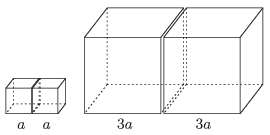(5 pont)

solution, statistics

P. 4719. A kayaker paddles up the river. The speed of the water is 5 m/s. At what speed should the kayaker paddle if he wants to cover a certain distance with the least work? (The drag force is proportional to the square of the speed.)

(5 pont)

solution, statistics

P. 4720. The operation of the demister is the following: a fan blows the air towards a cooled surface, and the condensed water vapour is collected in a container. How much heat is given off to the air in the room by the demister, rated at 180 W, in 15 hours, if it collected 4 litres of water? The temperature of the room is $\displaystyle 25~{}^\circ$C.

If the demister is considered to be a heat pump, what is its coefficient of performance (the ratio of the released heat to the electrical energy consumed)?

(4 pont)

solution, statistics

P. 4721. The working substance of a heat engine is a certain amount of monatomic ideal gas. The gas is taken through the cyclic process shown in the figure - which consists of two isobaric and two isochoric processes. What can the greatest thermodynamic efficiency of this engine be if in each cycle the useful work is the same as the internal energy of the gas at its initial state, which is $\displaystyle \frac32 p_0V_0$?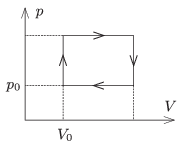(5 pont)

solution, statistics

P. 4722. There are two cylinders of cross section $\displaystyle A=2~\rm{dm}^2$ at rest on a frictionless, horizontal surface. The pistons of the cylinders can move easily and they are connected with a rigid rod. Each cylinder is connected to an unstretched spring. The values of the spring constant of the springs are $\displaystyle D_{\rm b}=24$ kN/m, and $\displaystyle D_{\rm j}=16$ kN/m. The volume of the air enclosed in the left, insulated cylinder is $\displaystyle V_{\rm 0b}=20~{\rm dm}^3,$ and that of in the right cylinder is $\displaystyle V_{\rm 0j}=30~{\rm dm}^3$, and the initial temperature of both gases is $\displaystyle T_0=300$ K. The temperature of the gas in the left cylinder is increased to $\displaystyle T_{\rm b}=1200$ K. The ambient air pressure is $\displaystyle p_0=10^5$ Pa.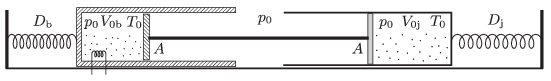$\displaystyle a)$ What is the displacement of the two cylinders and the piston with respect to the ground if the temperature of the gas in the right cylinder is kept at its initial value?

$\displaystyle b)$ What are the final values of the volume of the two samples of gas?

$\displaystyle c)$ How much heat was released by the heating element which heated the gas in the left cylinder?

(5 pont)

solution, statistics

P. 4723. Current $\displaystyle I$ in the wires shown in the figure flows in the direction of the arrows. (The wire forms two rays and two circular arcs of radius $\displaystyle r$ and of central angle $\displaystyle 90^\circ$.) What is the magnitude of the magnetic flux density $\displaystyle \boldsymbol B$ at point $\displaystyle O$ which is the common centre of the circles?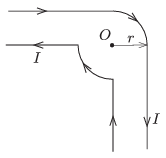(4 pont)

solution, statistics

P. 4724. The working temperature of the tungsten filament of an incandescent lamp rated at 230 V and 100 W is $\displaystyle 2400~{}^\circ$C. In an experiment the voltage applied to the lamp is gradually increased from zero, and it can be seen that the filament begins to glow at 17 V. What is the temperature and the resistance of the filament in this state, and how much power is dissipated in this state of the bulb?

We can assume that the emitted power is proportional to the fourth power of the temperature measured in Kelvins, the fifth power of the resistance is proportional to the sixth power of the absolute temperature and heat conduction is negligible.

(5 pont)

solution, statistics